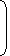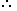# Tricks to solve partnership related questions in Aptitude Section1. Partnership:When two or more than two persons run a business jointly, they are called partners and the deal is known as partnership.
2. Ratio of Divisions of Gains:
1. When investments of all the partners are for the same time, the gain or loss is distributed among the partners in the ratio of their investments.Suppose A and B invest Rs. x and Rs. y respectively for a year in a business, then at the end of the year:(A’s share of profit) : (B’s share of profit) = x : y.
2. When investments are for different time periods, then equivalent capitals are calculated for a unit of time by taking (capital x number of units of time). Now gain or loss is divided in the ratio of these capitals.Suppose A invests Rs. x for p months and B invests Rs. y for q months then,(A’s share of profit) : (B’s share of profit)= xp : yq.
3. Working and Sleeping Partners:A partner who manages the the business is known as a working partner and the one who simply invests the money is a sleeping partner.
1. A and B invest in a business in the ratio 3 : 2. If 5% of the total profit goes to charity and A’s share is Rs. 855, the total profit is:
 A. Rs. 1425 B. Rs. 1500 C. Rs. 1537.50 D. Rs. 1576

Explanation:

Let the total profit be Rs. 100.

 After paying to charity, A’s share = Rs.95 x 3= Rs. 57. 5

If A’s share is Rs. 57, total profit = Rs. 100.

 If A’s share Rs. 855, total profit =100 x 855= 1500. 57
. A, B and C jointly thought of engaging themselves in a business venture. It was agreed that A would invest Rs. 6500 for 6 months, B, Rs. 8400 for 5 months and C, Rs. 10,000 for 3 months. A wants to be the working member for which, he was to receive 5% of the profits. The profit earned was Rs. 7400. Calculate the share of B in the profit.
 A. Rs. 1900 B. Rs. 2660 C. Rs. 2800 D. Rs. 2840

Explanation:

For managing, A received = 5% of Rs. 7400 = Rs. 370.

Balance = Rs. (7400 – 370) = Rs. 7030.

Ratio of their investments = (6500 x 6) : (8400 x 5) : (10000 x 3)

= 39000 : 42000 : 30000

= 13 : 14 : 10B’s share = Rs.7030 x 14= Rs. 2660. 37

#### A began a business with Rs. 85,000. He was joined afterwards by B with Rs. 42,500. For how much period does B join, if the profits at the end of the year are divided in the ratio of 3 : 1?

Correct! Wrong!

#### Simran started a software business by investing Rs. 50,000. After six months, Nanda joined her with a capital of Rs. 80,000. After 3 years, they earned a profit of Rs. 24,500. What was Simran's share in the profit?

Correct! Wrong!

#### A starts business with Rs. 3500 and after 5 months, B joins with A as his partner. After a year, the profit is divided in the ratio 2 : 3. What is B's contribution in the capital?

Correct! Wrong!

#### A and B entered into partnership with capitals in the ratio 4 : 5. After 3 months, A withdrew of his capital and B withdrew of his capital. The gain at the end of 10 months was Rs. 760. A's share in this profit is:

Correct! Wrong!

#### A and B started a partnership business investing some amount in the ratio of 3 : 5. C joined then after six months with an amount equal to that of B. In what proportion should the profit at the end of one year be distributed among A, B and C?

Correct! Wrong!

Quiz on Partnership
Great job